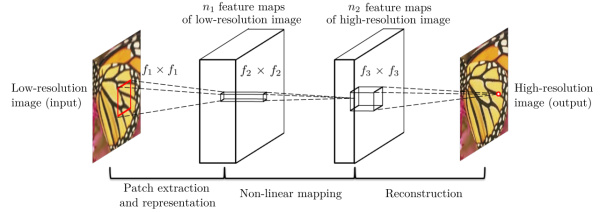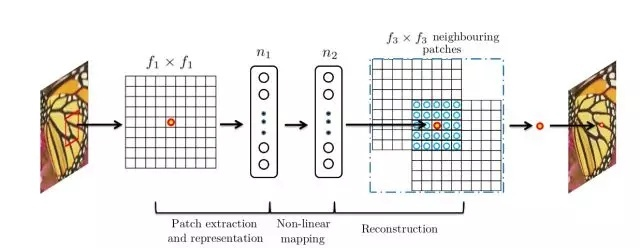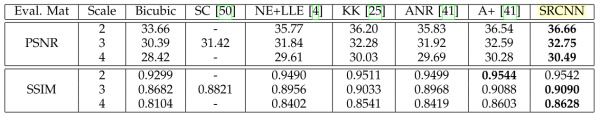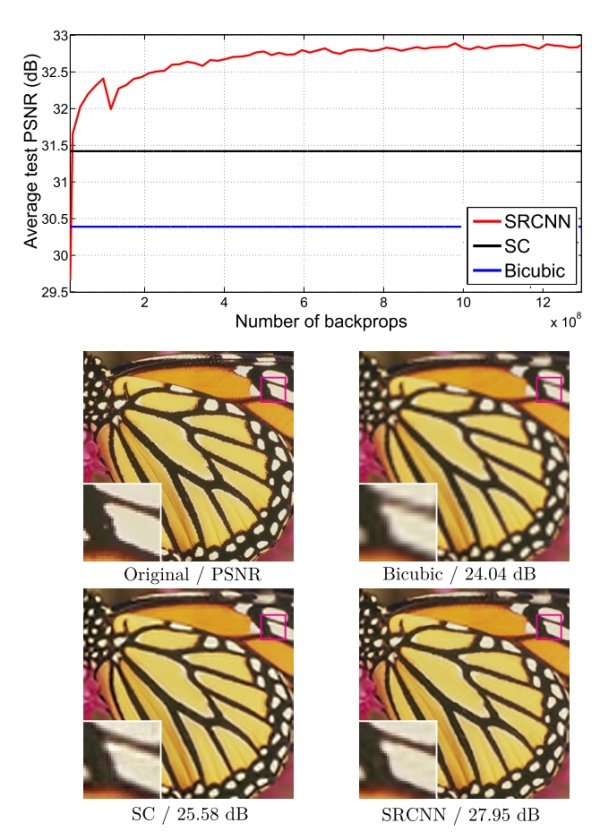## 1 概述

• 时间分辨率性能决定了视频输出的帧率，即实时效果；
• 空间分辨率性能决定了图像的画面清晰度究竟是720P，1080P，还是4K；
• 色阶分辨率性能决定了图像显示色彩的丰满程度与粒度

1. 预测型（prediction-based）
2. 边缘型（edge-based）
3. 统计型（statistical）
4. 图像块型（patch-based/example-based）

SR 也可分为两类:

1. 从多张低分辨率图像重建出高分辨率图像
2. 从单张低分辨率图像重建出高分辨率图像

SISR是一个逆问题，对于一个低分辨率图像，可能存在许多不同的高分辨率图像与之对应，因此通常在求解高分辨率图像时会加一个先验信息进行规范化约束

## 2 SRCNN

Super-Resolution Convolutional Neural Network
SRCNN, PAMI 2016, http://mmlab.ie.cuhk.edu.hk/projects/SRCNN.html

SRCNN是基于深度学习的超分辨重建领域的开山之作，继承了传统机器学习领域稀疏编码的思想，该方法对于一个低分辨率图像，先使用双三次（bicubic）插值将其放大到目标大小，再通过三层卷积网络做非线性映射，得到的结果作为高分辨率图像输出。

### 2.1 结构

1. 图像的图像块抽取与稀疏字典建立
2. 图像高、低分辨率特征之间的非线性映射
3. 高分辨率图像块的重建SRCNN的流程为：

1. 先将低分辨率图像使用双三次差值放大至目标尺寸（如放大至2倍、3倍、4倍），此时仍然称放大至目标尺寸后的图像为低分辨率图像(Low-resolution image)，即图中的输入(input)；

2. 将低分辨率图像输入三层卷积神经网络，

举例：在论文中的其中一实验相关设置，对YCrCb颜色空间中的Y通道进行重建，网络形式为

(conv1+relu1) $$\to$$ (conv2+relu2) $$\to$$ (conv3+relu3)

三个卷积层使用的卷积核的大小分为为 $$9\times 9$$ , $$1\times 1$$ 和 $$5\times 5$$ ，前两个的输出特征个数分别为 $$64$$ 和 $$32$$。

第一层卷积：卷积核尺寸 $$9×9\ (f_1×f_1)$$ ，卷积核数目 $$64\ (n_1)$$ ，输出64张特征图；
第二层卷积：卷积核尺寸 $$1×1\ (f_2×f_2)$$ ，卷积核数目 $$32\ (n_2)$$ ，输出32张特征图；
第三层卷积：卷积核尺寸 $$5×5\ (f_3×f_3)$$ ，卷积核数目 $$1\ (n_3)$$ ，输出1张特征图即为最终重建高分辨率图像。

SRCNN第二层卷积核尺寸为 $$n_1 \times 1 \times 1 \times n_2$$ ，以建立由低分辨率到高分辨率稀疏表示字典之间的非线性映射，输出的高分辨率稀疏字典的维度为 $$H_1 \times W_1 \times n_2$$ ，值得注意的是在这一步中SRCNN并未采用全连接层（fully connected layer）来进行特征图或是稀疏字典之间的映射，而是采用1x1卷积核，从而使得空间上每一个像素点位置的映射都共享参数，即每一个空间位置以相同的方式进行非线性映射；

SRCNN第三层卷积核尺寸为 $$n_2 \times f_3 \times f_3 \times C$$ ，由高分辨率稀疏字典中每一个像素点位置的 $$n_2 \times 1$$ 向量重建 $$f_3 \times f_3$$ 图像块，图像块之间相互重合覆盖，最终实现图片的超分辨率重建。1. 训练数据集：论文中某一实验采用91张自然图像作为训练数据集，对训练集中的图像先使用双三次差值缩小到低分辨率尺寸，再将其放大到目标放大尺寸，最后切割成诸多33×33图像块作为训练数据，作为标签数据的则为图像中心的21×21图像块（与卷积层细节设置相关）；

注：最原始的SRCNN输入不是低分辨率图像，而是低分辨率双立方插值后的图片

2. 损失函数：采用MSE函数作为卷积神经网络损失函数；

3. 卷积层细节设置：第一层卷积核 $$9×9$$ ，得到特征图尺寸为 $$(33-9)/1+1=25$$ ，第二层卷积核 $$1×1$$ ，得到特征图尺寸不变，第三层卷积核 $$5×5$$ ，得到特征图尺寸为 $$(25-5)/1+1=21$$ 。训练时得到的尺寸为 $$21×21$$ ，因此图像中心的 $$21×21$$ 图像块作为标签数据。（卷积训练时不进行padding）

### 2.2 重建效果